# PromQL函数

PromQL函数详解

### abs()¶

`abs(v instant-vector)` 返回输入向量中所有样本的绝对值。

``process_virtual_memory_max_bytes{job=~"prometheus"}``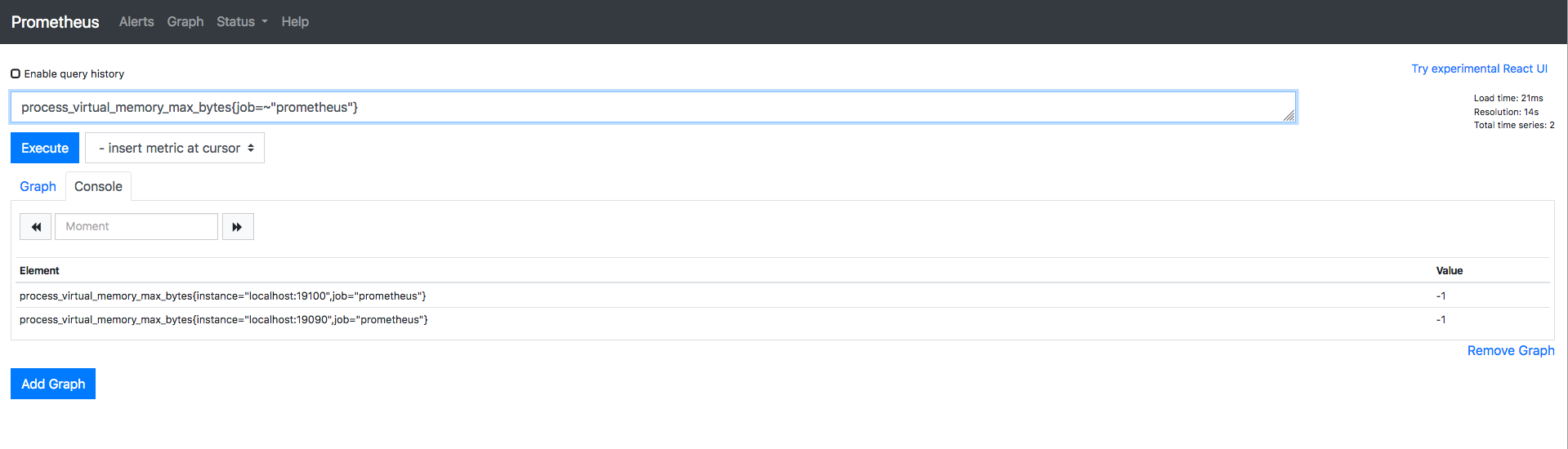``abs(process_virtual_memory_max_bytes{job=~"prometheus"})``### absent()¶

`absent(v instant-vector)`，如果输入向量中具有样本数据，则返回空向量；如果传递的瞬时向量中没有样本数据，则返回不带度量指标名称且带有标签的样本值为1的结果 当监控度量指标时，如果获取到的样本数据是空的， 使用 `absent` 方法对告警是非常有用的

``absent(process_virtual_memory_max_bytes{job=~"prometheus"})``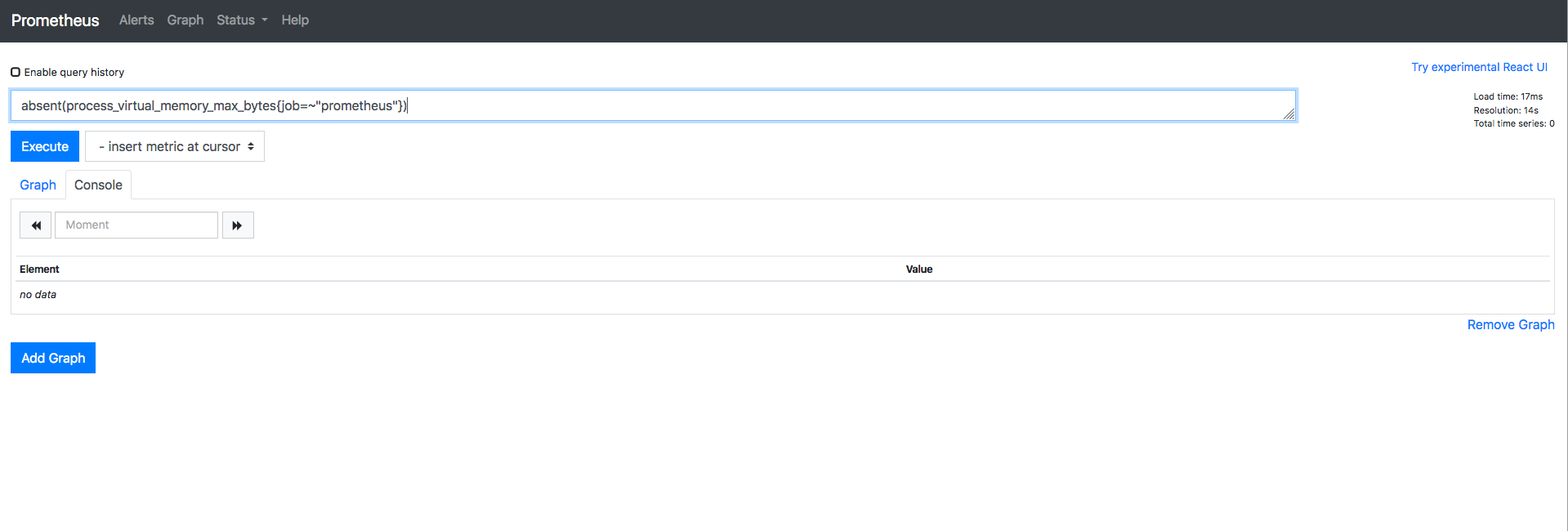``absent(process_virtual_memory_max_bytes{job=~"prometheus_1"})``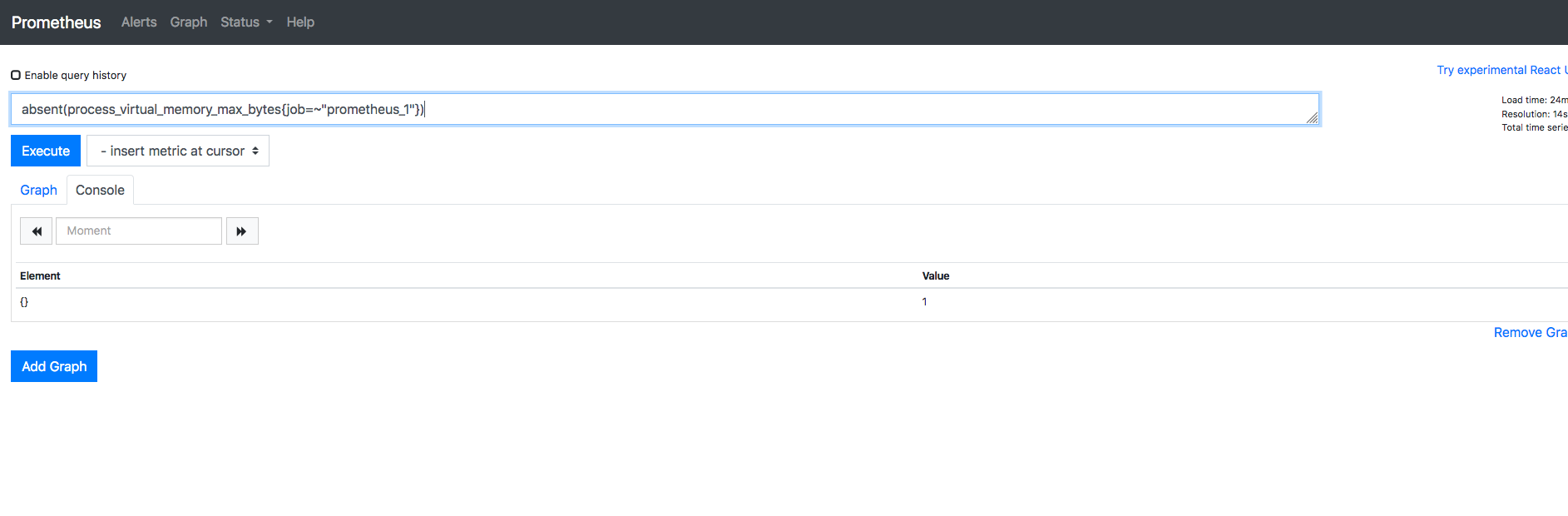### ceil()¶

ceil(v instant-vector) 向上舍入为最接近的整数。 示例中的values为0.18，那跟他最接近的整数是多少？答案是 `1`

``node_load5``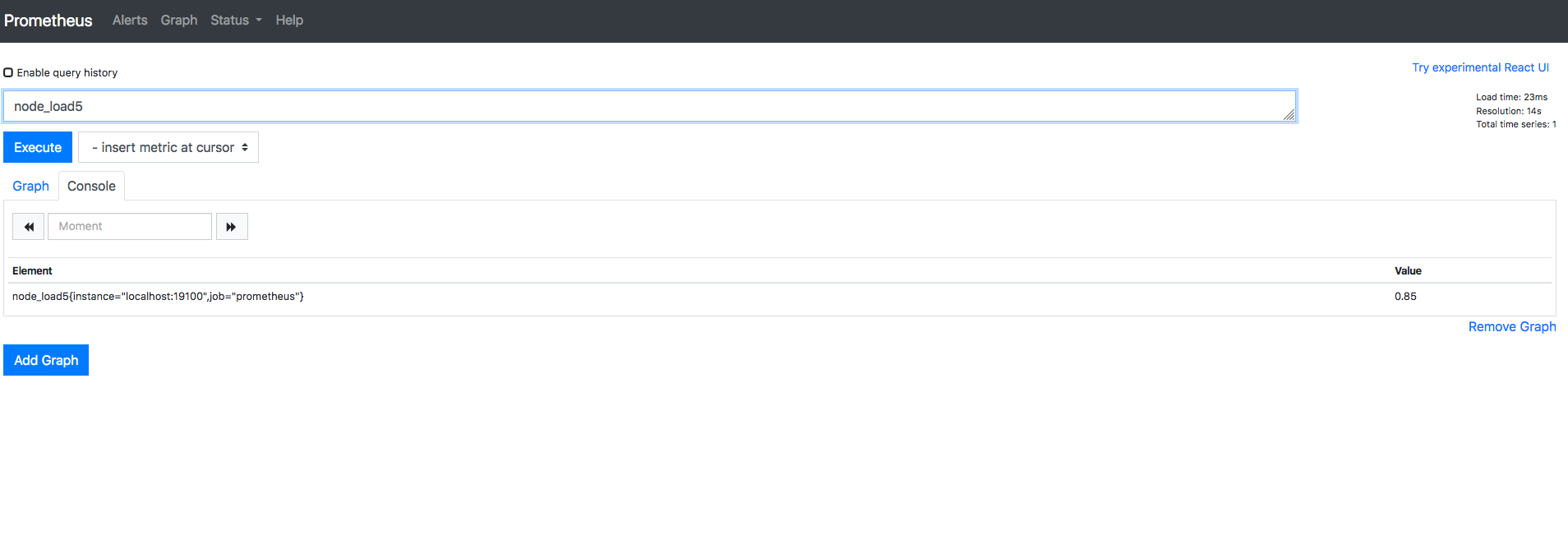``ceil(node_load5)``### changes()¶

`changes(v range-vector)` 输入一个区间向量， 返回这个区间向量内每个样本数据值变化的次数。

``node_load5[5m]``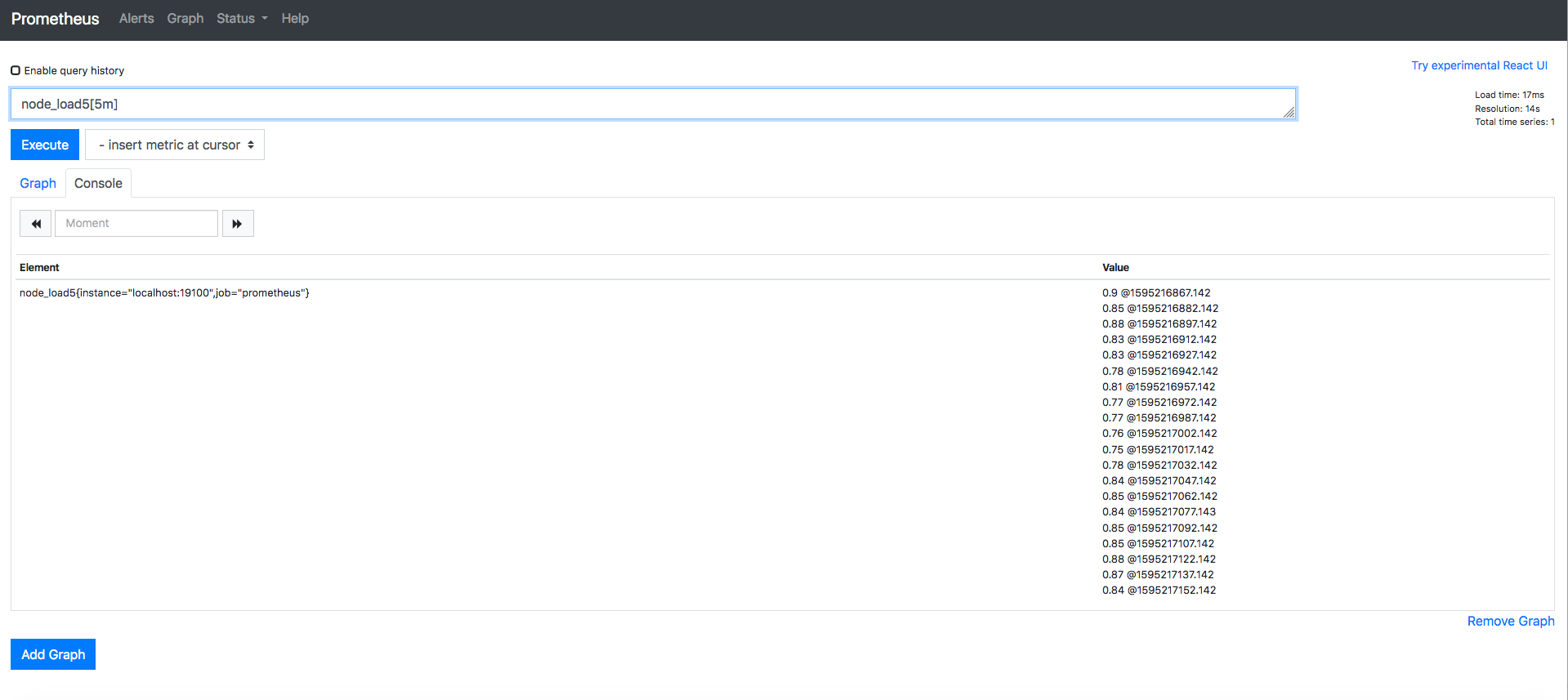``changes(node_load5[5m])``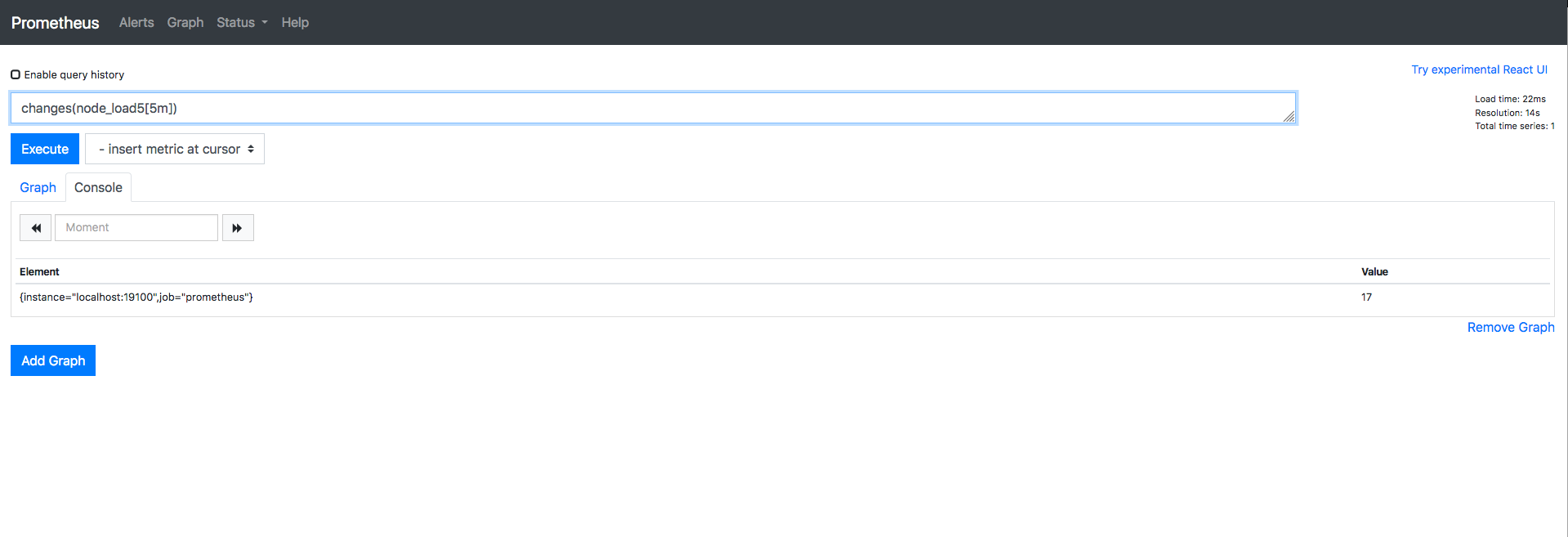### clamp_max()¶

`clamp_max(v instant-vector, max scalar)` 输入一个瞬时向量和最大值，样本数据值若大于max，则改为max，否则不变。

``sum(node_arp_entries)``

``clamp_max(sum(node_arp_entries),20)``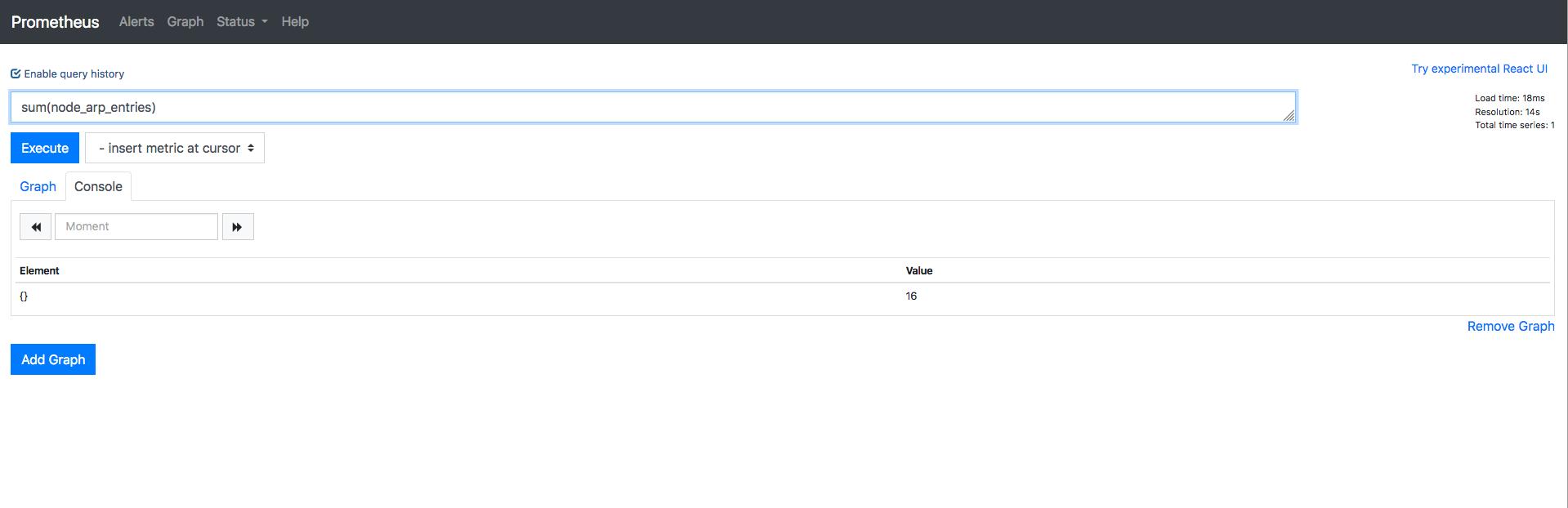``clamp_max(sum(node_arp_entries),10)``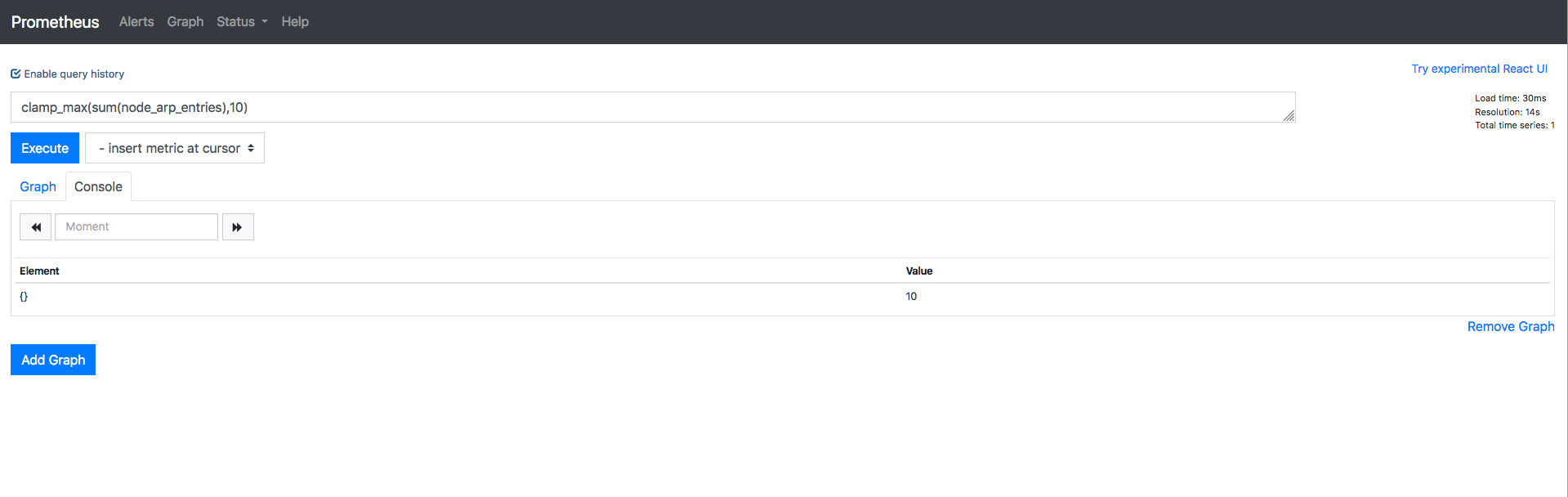### clamp_min()¶

clamp_min(v instant-vector)输入一个瞬时向量和最大值，样本数据值小于min，则改为min。否则不变。

max值大于瞬时值，显示max值

``clamp_min(sum(node_arp_entries),20)``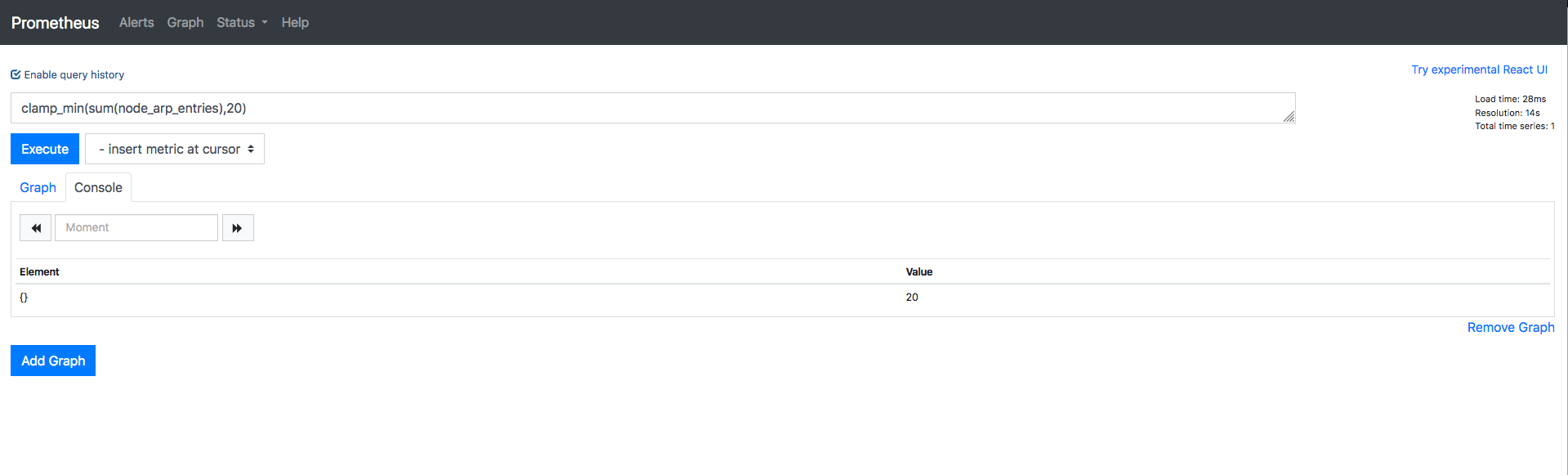max值小于瞬时值，显示瞬时值

clamp_min(sum(node_arp_entries),10)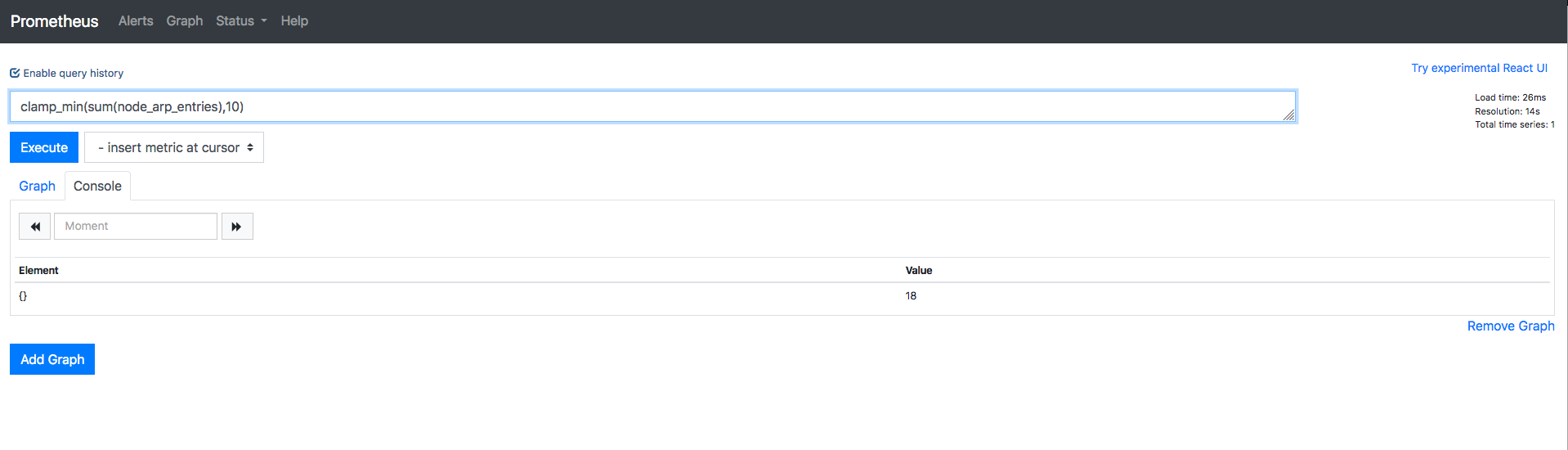### scalar()¶

scalar(v instant-vector) 输入只有一个元素的瞬时向量，返回（key:value）=（”scalar”: 样本数值）。如果输入向量有多个元素，scalar则返回NaN。

``scalar(node_arp_entries{device="ens18",instance="localhost:19100",job="prometheus"})``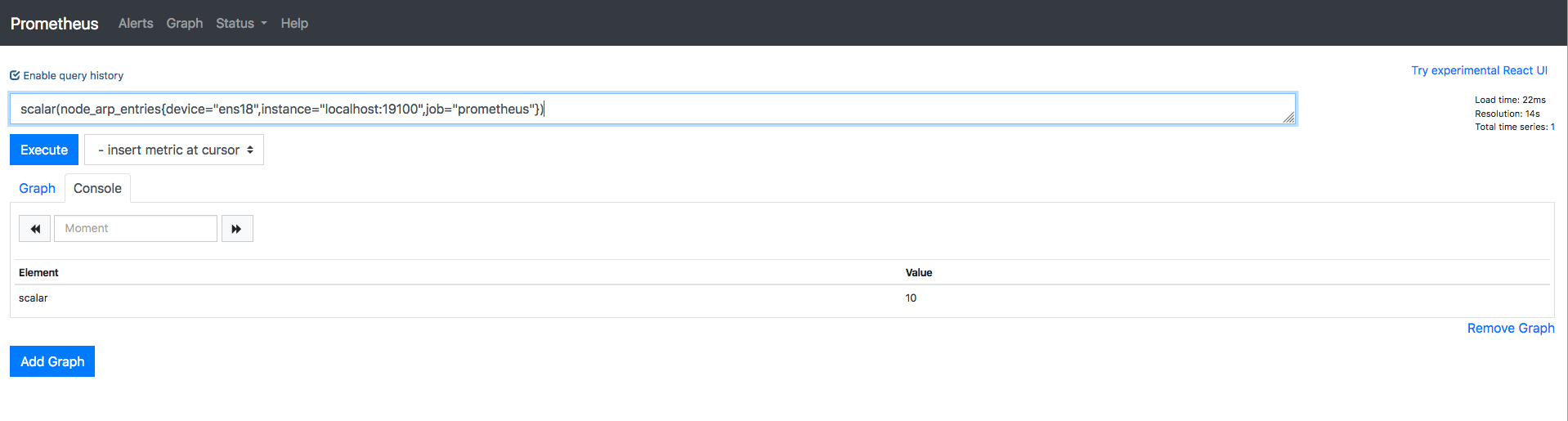``scalar(node_arp_entries)``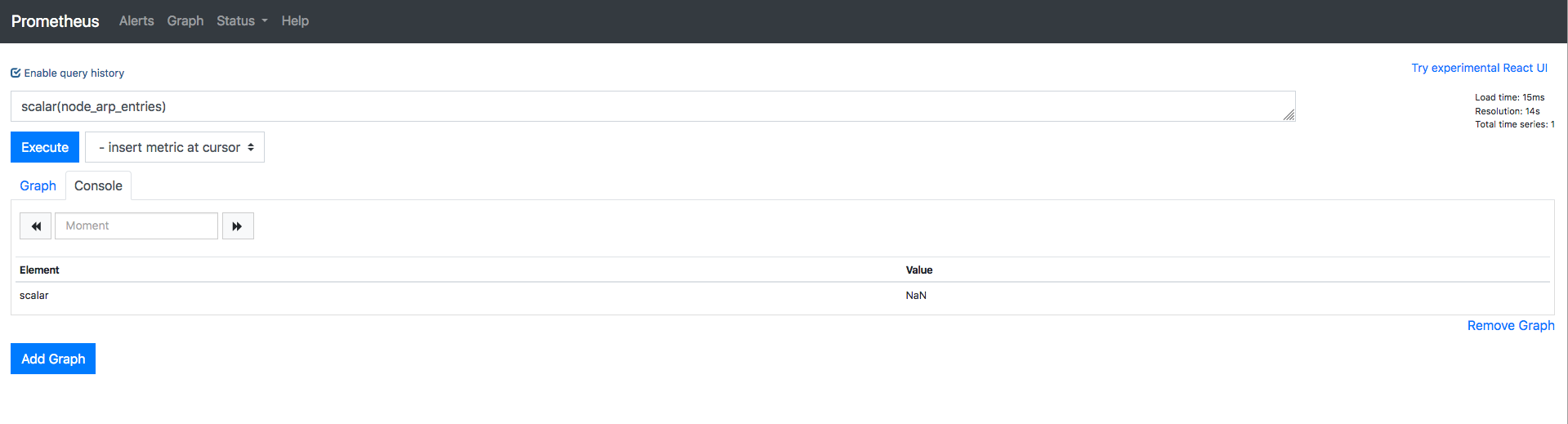### day_of_month()¶

day_of_month(v=vector(time()) instant-vector)返回给定UTC时间所在月的第几天。返回值范围：1~31。

``day_of_month(vector(time()))``

### day_of_week()¶

day_of_week(v=vector(time()) instant-vector)返回给定UTC时间所在周的第几天。返回值范围：0~6. 0表示星期天。 跟上面一样的意思，今天是周一的话，返回的值则是1。

``day_of_week(vector(time()))``

### days_in_month()¶

days_in_month(v=vector(time()) instant-vector)返回当月一共有多少天。返回值范围：`28~31`.

``days_in_month(vector(time()))``

### delta()¶

delta(v range-vector)的参数是一个区间向量，返回一个瞬时向量。它计算一个区间向量 v 的第一个元素和最后一个元素之间的差值。由于这个值被外推到指定的整个时间范围， 所以即使样本值都是整数，你仍然可能会得到一个非整数值。例如，下面的例子返回过去两小时的 CPU 温度差：

`delta(cpu_temp_celsius{host="zeus"}[2h])` 这个函数一般只用在 Gauge 类型的时间序列上。

``node_cpu_seconds_total{cpu='0',mode="idle"}[1h]``

``delta(node_cpu_seconds_total{cpu='0',mode="idle"}[1h])``

### deriv()¶

deriv(v range-vector)计算一个区间向量v中各个时间序列二阶导数，使用简单线性回归 deriv二阶导数返回值类型只能是gauges。

(简单来说，一阶导数是自变量的变化率，二阶导数就是一阶导数的变化率，看其变化的快慢，也就是一阶导数变化率的变化率。)

``deriv(node_cpu_seconds_total{cpu='0',mode='idle'}[5m])``

### exp()¶

exp(v instant-vector)输入一个瞬时向量, 返回各个样本值的e指数值，即为e的N次方。特殊情况如下所示：

`Exp（+ Inf）= + Inf` `+Inf` 是最高bucket的上限值，所以xxx_bucket是所有采样点的数量，是Prometheus自动增加的一个bucket。

``exp(node_cpu_seconds_total{cpu='0',mode="idle"})``

`Exp（NaN）= NaN` 此时返回的值不代表聚合运算工作不正常，prometheus查询语句中分母值为0时，语句返回值会为NaN。因此，你不能把对比率加起来，这个比率其实就是类似于成人与儿童之比率是1比6。

``histogram_quantile(0.9, rate(prometheus_http_request_duration_seconds_bucket[10m])>0)``

``````sum by (job, handler)(rate(prometheus_http_request_duration_seconds_sum[5m])>0)
/
sum by (job, handler)(rate(prometheus_http_request_duration_seconds_count[5m])>0)``````

### floor()¶

floor(v instant-vector)函数与 ceil() 函数相反，将 v 中所有元素的样本值向下四舍五入到最接近的整数。 很简单的一个函数，向下取最接近的整数。

``floor(node_cpu_seconds_total{cpu='0',mode='user'})``

### histogram_quantile()¶

histogram_quantile(φ float, b instant-vector) 从 bucket 类型的向量 b 中计算 φ (0 ≤ φ ≤ 1) 分位数（百分位数的一般形式）的样本的最大值。（有关 φ 分位数的详细说明以及直方图指标类型的使用，请参阅直方图和摘要。 向量 b 中的样本是每个 bucket 的采样点数量。每个样本的 labels 中必须要有 le 这个 label 来表示每个 bucket 的上边界，没有 le 标签的样本会被忽略。直方图指标类型自动提供带有 _bucket 后缀和相应标签的时间序列。 可以使用 rate() 函数来指定分位数计算的时间窗口。 例如，一个直方图指标名称为 prometheus_http_request_duration_seconds_bucket，要计算过去 10 分钟内 第 90 个百分位数，请使用以下表达式：

``histogram_quantile(0.9, rate(prometheus_http_request_duration_seconds_bucket{handler="/api/v1/query"}[10m]))``

``histogram_quantile(0.9, sum(rate(prometheus_http_request_duration_seconds_bucket[10m])) by (job, le))``

histogram_quantile 这个函数是根据假定每个区间内的样本分布是线性分布来计算结果的也就是说，

### holt_winters()¶

holt_winters(v range-vector, sf scalar, tf scalar)基于区间向量v，生成事件序列数据平滑值。平滑因子sf越低, 对老数据越重要。 趋势因子tf越高，越多的数据趋势应该被重视0<sf,tf<=1。 holt_winters仅用于gauges。

### hour()¶

hour(v=vector(time()) instant-vector) 函数返回被给定 UTC 时间的当前第几个小时，时间范围：0~23。

``hour(vector(time()))``

### idelta()¶

idelta(v range-vector)输入一个区间向量，返回（key: value ）=（ 度量指标： 每最后两个样本值差值）。

``node_cpu_seconds_total{cpu='0',mode="idle"}[10m]``

### increase()¶

increase(v range-vector)输入一个区间向量，返回：（key:value） = （度量指标：last值-first值），函数获取区间向量中的第一个和最后一个样本并返回其增长量, 它会在单调性发生变化时(如由于采样目标重启引起的计数器复位)自动中断。 由于这个值被外推到指定的整个时间范围，所以即使样本值都是整数，你仍然可能会得到一个非整数值。

``nginx_server_requests{host="*",job="nginx-vts",code='total'}[5m]``

``increase(nginx_server_requests{host="*",job="nginx-vts",code='total'}[5m])``

increase 的返回值类型只能是计数器类型，也就是counter，主要作用是增加图表和数据的可读性。使用 rate 函数记录规则的使用率，以便持续跟踪数据样本值的变化。

### irate()¶

irate(v range-vector) 函数用于计算区间向量的增长率，但是其反应出的是瞬时增长率。irate 函数是通过区间向量中最后两个两本数据来计算区间向量的增长速率，它会在单调性发生变化时(如由于采样目标重启引起的计数器复位)自动中断。 这种方式可以避免在时间窗口范围内的“长尾问题”，并且体现出更好的灵敏度，通过irate函数绘制的图标能够更好的反应样本数据的瞬时变化状态。

``nginx_server_requests{host="*",job="nginx-vts",code='total'}[5m]``

irate只能用于绘制快速移动的计数器。在长期趋势分析或者告警中更推荐使用 rate 函数。因为使用 irate 函数时，速率的简短变化会重置 FOR语句，形成的图形有很多波峰，难以阅读。

### rate()¶

`label_join(v instant-vector, dst_label string, separator string, src_label_1 string, src_label_2 string, ...)`

rate（v range-vector） 可以直接计算区间向量 v 在时间窗口内平均增长速率，它会在单调性发生变化时(如由于采样目标重启引起的计数器复位)自动中断。该函数的返回结果不带有度量指标，只有标签列表。

rate() 函数返回值类型只能用计数器，在长期趋势分析或者告警中推荐使用这个函数。

`当将 rate() 函数与聚合运算符（例如 sum()）或随时间聚合的函数（任何以 _over_time 结尾的函数）一起使用时，必须先执行 rate 函数，然后再进行聚合操作，否则当采样目标重新启动时 rate() 无法检测到计数器是否被重置。`

### label_join（）¶

``````up{endpoint="https",instance="192.168.1.210:6443",job="apiserver",namespace="default",service="kubernetes"}

label_join(up{endpoint="https",instance="192.168.1.210:6443",job="apiserver",namespace="default",service="kubernetes"}, "join_test", ",", "job", "service")``````

``````kube_pod_container_resource_limits{container=~"grafana"}
# lable_join
label_join(kube_pod_container_resource_limits{container=~"grafana"},"pod_name","_","pod","container")``````

### label_replace（）¶

`label_replace(v instant-vector, dst_label string, replacement string, src_label string, regex string)`

`label_replace(up, "host", "\$1", "instance", "(.*):.*")`

``````up{host="localhost",instance="localhost:8080",job="cadvisor"}   1
up{host="localhost",instance="localhost:9090",job="prometheus"}   1
up{host="localhost",instance="localhost:9100",job="node"}   1``````

``label_replace(up,"host","6666","service","(.*)-.*-.*")``

``````up{endpoint="http-metrics",host="6666",instance="192.168.1.210:10252",job="kube-controller-manager",namespace="kube-system",pod="kube-controller-manager-k8s-m1",service="kube-controller-manager"}
up{endpoint="https-self",host="6666",instance="10.240.36.115:9443",job="kube-state-metrics",namespace="monitoring",pod="kube-state-metrics-dbb85dfd5-8xtdh",service="kube-state-metrics"}``````

### predict_linear()¶

predict_linear(v range-vector,t scalar) 基于区间向量，使用简单的线性回归时间序列 t 秒的值，从而可以对时间序列的变化趋势做出预测。例如，根据过去2小时的时间样本数据，预测主机的可用磁盘空间在8小时内是否会被占满。

``predict_linear(node_filesystem_free_bytes{job="prometheus",mountpoint="/"}[2h], 8 * 3600 ) / 1024 / 1024``

### ln（）¶

ln(v instance-vector)计算瞬时向量v中所有样本数据的自然对数。特殊情况：

``````ln(+Inf) = +Inf
ln(0) = -Inf
ln(x < 0) = NaN
ln(NaN) = NaN``````

### log2（）¶

log2(v instant-vector) 函数计算瞬时向量v中所有样本数据以2为底的对数。特殊情况同上。

### log10（）¶

log10(v instant-vector)函数计算瞬时向量v中所有样本数据以10为底的对数。相当于ln()。特殊情况同上。

### minute（）¶

minute(v=vector(time()) instant-vector)返回给定UTC时间当前小时的第多少分钟。结果范围：0~59。

`minute(vector(time()))`

### month（）¶

month(v=vector(time()) instant-vector)返回给定UTC时间当前属于第几个月，结果范围：0~12。

`month(vector(time()))`

### predict_linear（）¶

predict_linear（v range-vector，t scalar） 函数可以预测时间序列 v 在 t 秒后的值。它基于简单线性回归的方式，对时间窗口内的样本数据进行统计，从而可以对时间序列的变化趋势做出预测。该函数的返回结果不带有度量指标，只有标签列表。

`predict_linear(node_filesystem_files_free{fstype="ext4"}[2h], 4 * 3600) < 0`

`predict_linear(nginx_server_requests{code="total",host="*",instance="192.168.1.220:9913"}[5m],10*6)`

### resets（）¶

resets(v range-vector) 的参数是一个区间向量。对于每个时间序列，它都返回一个计数器重置的次数。两个连续样本之间的值的减少被认为是一次计数器重置。 这个函数一般只用在计数器类型的时间序列上。

### round（）¶

round(v instant-vector, to_nearest=1 scalar) 函数与 ceil 和 floor 函数类似，返回向量中所有样本值的最接近的整数。to_nearest 参数是可选的,默认为 1,表示样本返回的是最接近 1 的整数倍的值。你也可以将该参数指定为任意值（也可以是小数），表示样本返回的是最接近它的整数倍的值。

`node_cpu_seconds_total{cpu="0",instance="localhost:19100",job="prometheus",mode="idle"}`

`round(node_cpu_seconds_total{cpu="0",instance="localhost:19100",job="prometheus",mode="idle"})`

### sort（）¶

sort(v instant-vector) 函数对向量按元素的值进行升序排序，返回结果：key: value = 度量指标：样本值`[升序排列]`

`sort(node_cpu_seconds_total)`

### sort_desc（）¶

sort_desc(v instant-vector) 函数对向量按元素的值进行降序排序，返回结果：key: value = 度量指标：样本值`[降序排列]`

`sort_desc(node_cpu_seconds_total)`

### sqrt（）¶

sqrt(v instant-vector) 函数计算向量 v 中所有元素的平方根。

`sqrt(node_cpu_seconds_total)`

### time（）¶

time() 函数返回从 1970-01-01 到现在的秒数。注意：它不是直接返回当前时间，而是时间戳。

### timestamp（）¶

timestamp（v instant-vector）返回给定向量的每个样本的时间戳，作为自1970年1月1日UTC以来的秒数。

timestamp(node_cpu_seconds_total)

### vector（）¶

vector(s scalar)函数，返回：（key: value）= （{}, 传入参数值）。

vector(1) vector(time())

### year（）¶

year（v = vector（time（））instant-vector）返回UTC中每个给定时间的年份。

### _over_time()¶

• avg_over_time(range-vector) : 区间向量内每个度量指标的平均值。

• min_over_time(range-vector) : 区间向量内每个度量指标的最小值。

• max_over_time(range-vector) : 区间向量内每个度量指标的最大值。

• sum_over_time(range-vector) : 区间向量内每个度量指标的求和。

• count_over_time(range-vector) : 区间向量内每个度量指标的样本数据个数。

• quantile_over_time(scalar, range-vector) : 区间向量内每个度量指标的样本数据值分位数，φ-quantile (0 ≤ φ ≤ 1)。

• stddev_over_time(range-vector) : 区间向量内每个度量指标的总体标准差。

• stdvar_over_time(range-vector) : 区间向量内每个度量指标的总体标准方差。